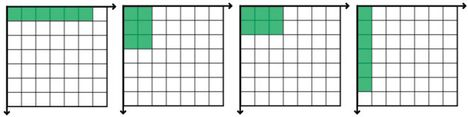# Practice on Toph

Participate in exhilarating programming contests, solve unique algorithm and data structure challenges and be a part of an awesome community.

# Magician's Offer!

By Ariful_Efath · Limits 2s, 512 MB

Rosi Vidmun is a greedy landlord who likes to grab as much land as he can. Currently he has $P$ square km land and he wants more! Nobody likes Rosi, but he doesn’t bother about it. Yesterday, a Magician came to him and gave him an offer of giving a larger land. He will get a land of $P^X$ square km, but only if he is able to solve a puzzle. Magician has a land having Infinite length and width. Rosi is free to select any length and width from the top left corner of the land. He needs to answer how many possible ways are there to make $P^X$ square km land where the length and width must be a positive integer. If he takes the offer and fails to answer, the magician will take all of his land. Rosi was confused to take the offer. So, the magician told him, he will count the answer as correct if Rosi could tell the last 7 digits of the actual answer. As 7 is a lucky number, Rosi took the offer and thought that it will bring luck to him. I know you might also have started hating Rosi! But, help him to find the answer he needs. He might give you 10% of the land!

Rosi is assuming that the magician might change the value of $P$ and $X$ before asking him the answer. So, you have to solve it for different values of $P$ and $X$.

## Input

Input starts with a positive integer $T$ ($≤ 1000$) denoting the number of test cases.

Each case starts with a line containing two integers $P$ and $X$ where $1 ≤ P ≤ 10^{12}$ and $1 ≤ X ≤ 10^5$.

## Output

Output should consist of exactly $T$ lines, one for each test case. Each line should be of the format "Case X: Y" (without the quotes), where $X$ is the test case and $Y$ is the last 7 digits of the answer that Rosi needs. If the answer has less than 7 digits, print it with leading zeros.

## Sample

InputOutput
3
6 1
7 2
30 300

Case 1: 0000004
Case 2: 0000003
Case 3: 7270901


For $P=6$ and $X=1$, Rosi has the following ways to select his land.uDebug

### Statistics

82% Solution Ratio

forthright48Earliest, Feb '16

tuhin107494Fastest, 0.2s

kfoozminusLightest, 1.3 MB

steinumShortest, 729B

### Submit

 Intra NSU Programming Contest 2016Feb '16 Intra NSU Programming Contest 2016 (Semi-live)Feb '16